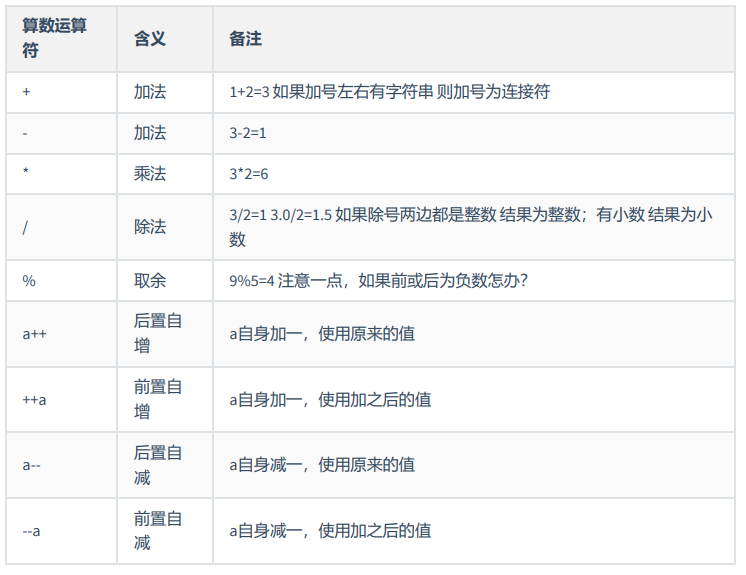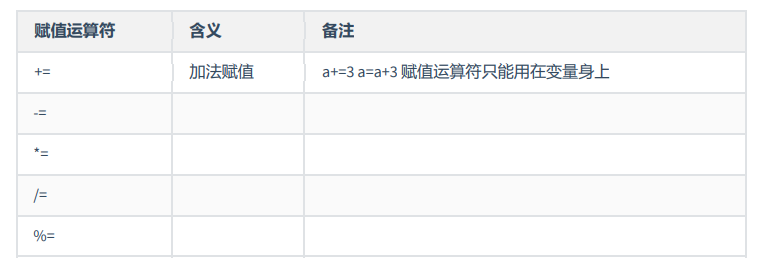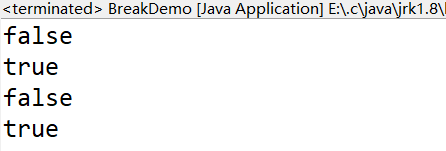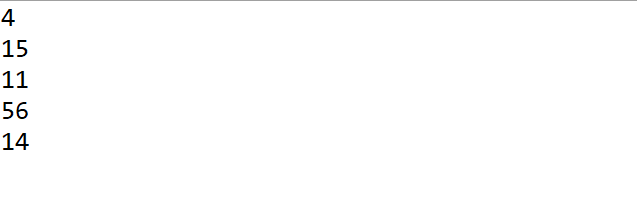• 算术运算符 a = 4 b = 7 print('a + b =',a + b) # 相加 print('a - b =',a - b) # 相减 print('a * b =',a * b) # 相乘 print('a / b =',a / b) # a除以b print('a % b =',a % b) # 取余 公式 c = a - b * ...

目录

算术运算符

比较运算符

赋值运算符

算术运算符

a = 4
b = 7

print('a + b =',a + b) # 相加

print('a - b =',a - b) # 相减

print('a * b =',a * b) # 相乘

print('a / b =',a / b) # a除以b

print('a % b =',a % b) # 取余 公式 c = a - b * (a // b)

print('a ** b =',a ** b) # a的b次方

print('a // b =',a // b) # a除以b后，向下取整

+可以用于连接字符串，*可以用于字符串重复

c = 'Pyt'
d = 'hon'

print(c)
print(d)
print(c + d)
print(c+d*4)

比较运算符

结果返回布尔值

a=4
b=7

print(a == b)  # a，b是否相等
print(a != b)  # a，b是否不相等
print(a > b)   # a是否大于b
print(a < b)   # a是否小于b
print(a >= b)  # a是否大于等于b
print(a <= b)  # a是否小于等于b

赋值运算符

将结果赋值给变量

a = 4
b = 7

#a+b的结果并赋值给c
c = a + b
print(c)

#c+a并赋值给c
c += a
print(c)

#c-a并赋值给c
c -= a
print(c)

#c*a并赋值给c
c *= a
print(c)

#c/a并赋值给c
c /= a
print(c)

#c%a并赋值给c
c %= a
print(c)

#c**a并赋值给c
c **= a
print(c)

#c//a并赋值给c
c //= a
print(c)

展开全文• JS算术运算符赋值运算符比较运算符逻辑运算符运算符优先级小例子拓展-位运算操作不合法标签属性（自定义标签属性）CSS选择器补充 你好！ 这是你第一次使用 Markdown编辑器 所展示的欢迎页。如果你想学习如何使用...

你好！ 这是你第一次使用 Markdown编辑器 所展示的欢迎页。如果你想学习如何使用Markdown编辑器, 可以仔细阅读这篇文章，了解一下Markdown的基本语法知识。

算术运算符

算术运算符不能用小数做运算，要先把小数转整数，再除相对应的倍数，以下是几种算术运算符
加—————[+]
减—————[-]
乘—————[*]
除—————[ / ]
取模、取余 —[%]

1. 隐式类型转换
+ 在有字符串的时候,会进行字符串拼接
- * / % 会尽力把不是数字的转化为数字，如果两边有任意一边有不是字符串数字 就会 出现NaN
布尔值和数字运算会转换成0(false)和1(ture) 运算
false + 8 = 8
除number string boolean 外，讨论其他对象和另一个对象的加法无意义

%：

10%3 = 1
取余数
5.5%2 = 1.5
取余数 1.5
%会有小数

++：

let a = 10
console.log(a++) //10   后置自增表达式还是原来的值
console.log(++a) //11  前置自增先自增

取表达式整体时才有区别

let a = 10
let b = 5

–：

let a = 10
a--//9

意思和++一样
隐式转换
++或-- 会强行转数字进行运算，返回值也是数字

1. NaN ——————————–not a number(不是一个数字)
不是数字的数字类型（number类型）
NaN和自己都不相等
isNaN( obj ) 判断是否为NaN,是返回true,否返回false;

2. 显示类型转化

3. 模板字符串

let oWrap = document.getElementById("wrap")
let x = "啦啦啦啦"
oWrap.innerHTML = "<p></p>"字符串不能换行
oWrap.innerHTML = "<p"+ x +
"</p>"

用+拼接
单引号或双引号描述的字符串内部不能换行，但是模板字符串 可以  (在键盘左上角Esc下面)

oWrap.innerHTML = <p>
<a>${x}</a> </p> 想显示$，用\转义符（转义只存在字符串或正则里）

oWrap.innerHTML = <p>
<a>\\${x}</a>
</p>

赋值运算符

let a = 20
a+=5 //a = a + 5
<div  id="wrap">
<p id="goudan"></p>
</div>

let oWrap = document.getElementById("wrap")
let oP = document.getElementById("goudan")
oWrap.innerHTML = oWrap.innerHTML + "<a>1234</a>"
oP.innerHTML = "789"
console.log(oWrap) //有p标签，但是标签里不会加文字

或者在改变oWrap 以后再获取oP，就可以获取到了
+=
赋值后再改变这个标签，会找不到之前的标签
也可以在不影响以前内容，添加标签

=
let a = 5
a -=2 //a = a - 2 //3
a
=3 //a = a * 3 //
a/=6 //a = a / 5 //
a %= 5 //a = a % 5 //0
a%5 结果不可能超过5，不可能超过被磨的那个数

比较运算符

比较运算符，得到的结果都是布尔值
= 赋值运算符
== 只比较值是否相等，不会管数据类型
=== 值和数据类型都要一样，先会判断数据类型，再判断值
如果不是特别有必要，（模糊比较）否则都用===判断，===效率更快

!= 不等
!== 不全等

let a = 10
let b = 10
console.log( a == b)//true
console.log(a === b)//true

let a = 10
let b = “10”
console.log( a == b)//true
console.log(a === b)//false

比较指针

let a = 
let b = 
console.log( a == b)//false
console.log(a === b)//false

基础数据类型（栈内存）
string number boolean undefined null symbol

引用数据类型（堆内存）
boject
[]{}
引用数据类型在做判断时，是比较指针指向

不要用基础数据类型和引用数据类型作比较，无意义

> < >= <=

let a = 10
let b = 50
console.log(a > b)//false

let a = "3"
let b = "20"
console.log(a > b)//true

字符串比较是通过ascll码来比较的
判断结果是boolean值

逻辑运算符

与—————[&&]
或—————[ || ]
非—————[！]

1. && 遇到假停
let a = true && false; 只考虑布尔值时，真真为真，其他为假，两边都为真，才是真
console.log(a)//false
真正的运用：遇到假就停，取这个假值，否则取最后的值
假的意思：数据被强行转成布尔值，是真是假

哪些数据在被转成布尔值的时候是 false:
0 undefined null false ‘’ NaN
取数值本身，不会取布尔值

let a = '' && 8 && 9
console.log(a)//''
let a = 2 && 7 && 9
console.log(a)//9

1. || 遇到真停
let a = true || false; 只考虑布尔值时，假假为假，两边有一个为真，就是真
真正的运用：遇到真就停，取真值，否则取后面的值
let a = 4 || 0 || NaN
console.log(a) //4

let a = '' || 0 || NaN
console.log(a) //NaN

1. 只考虑布尔值时，取反
真正的作用：取数据对应相反的布尔值
非运算完一定是一个布尔值结果
let a = !0
console.log(a)//true

let a = !!0
console.log(a)//false  //如果想知道这个数据对应的是真值还是假值，就可以用！！
取某一个数据对应的布尔值

1. , 运算符
let a = (4,5,6)

运算符优先级

从等级最高依次降低等级
. [] () 等级最高
++ – ! typeof
* / %
+ -
> < >= <=
&&
||
三目 ？：
=
, 等级最低

小例子

//例子1：
let a = 8 || 9 && 0
a = 8

//例子2：
let a = 10,
let b= 5
a = 8 || (b=6)
console.log(a)//8
console.log(b)//5

//例子3：
let a = 8 || 5 && (b=6)
console.log(a)//8
console.log(b)//5

从最惰性的开始解析，有近路可以走，就直接走近路了
惰性运算，按最低优先级开始判断是否有必要进行运算

拓展-位运算

二进制 逢二进一 0 1 2 3 4
0 1 10 11 100

let a = 15
15的二进制
00000000 00000000 00000000 00001111 （28个0)共32位）
第一位代表符号位，0代表正数

-15的二进制
00000000 00000000 00000000 00001111 先取15的二进制
11111111 11111111 11111111 11110000 取反码
11111111 11111111 11111111 11110001 反码+1

按位取非~
let a = 7
let b = ~a
b是个十进制
先求a的二进制
00000000 00000000 00000000 00000111
再按位取非
11111111 11111111 11111111 11111000 这就是b的值

得到b的十进制数
11111111 11111111 11111111 11110111 减1
00000000 00000000 00000000 00001000 取反码
b = -8

按位与 是两个数之间的操作
let a = 10
let b = 8
let c = a&b

00000000 00000000 00000000 00001010 10的二进制码
00000000 00000000 00000000 00001000 8的二进制码

操作不合法标签属性（自定义标签属性）

还可以操作合法标签属性，一般不会用他们操作合法标签属性，合法标签属性可以直接·操作，更快
1、getAttribute() 拿属性
2、setAttribute() 设置属性
3、removeAttribute() 移除属性

<div id="wrap" one="789"></div>
cument.getElementById("wrap")

1. 获取自定义标签属性
console.log(oWrap.getAttribute("one"))	//获取到自定义标签one 的值789

1. 设置自定义标签属性
console.log(oWrap.setAttribute("one","123"))//第一个值是要设置的自定义标签，第二个值是修改后的值
console.log(oWrap.setAttribute("two","1234"))也可以新增一个自定义属性

自定义属性的作用：
框架最根本的原理就是基于自定义标签属性去操作的，可以把一些信息，存在自定义标签里，在HTML里存储简单信息的一种方式
vue，angular框架，都是基于自定义的属性去操作的，解析标签的自定义属性，从而把他变成对应的dom结构

1. 移除自定义标签属性
console.log(oWrap.removeArribute("one"))

1. 获取标签的内容
<div id="wrap">123456789</div>
let oWrap = document.getElementById("wrap")
console.log(oWrap.innerHTML)//123456789

.innerHTML 可以解析标签
-innerText 不可以解析标签，会显示标签 火狐低版本没有，需要用.textContent
input用innerHTML获取不到里面的文字，要用.value

<input type="text" id="inp">
<button id="btn"></button>
let oInp = document.getElementById("inp")
let oBtn = document.getElementById("btn")
document.oBtn.onclick = function(){
console.log(oInp.innerHTML)//不能获取input框里的文字
console.log(oInp.value)//这样才可以获取到input框里的文字
}

CSS选择器补充

div#wrap.on.goudan{   //首先满足是个div ，id是wrap ，class名是on和狗蛋
width:100px;
height:100px;
background-color:pink;
}
<div id="wrap" class="on goudan"></div>

展开全文• 算术运算符

目录

一、算术运算符

二、赋值运算符

三、比较运算符

四、逻辑运算符

五、 位运算符

六、三目运算符

一、算术运算符public class Sample {
public static void main(String[] args) {
/*
a++
1.先开辟临时存储区 将a的值赋值进去
2.a自身加一
3.临时存储区的值等待被调用 输出 赋值 参与运算
*/
int i = 0;
i++;
System.out.println(i++); //1
System.out.println(i); //2
int j = i++;
System.out.println(i); //3
System.out.println(j); //2
i = i++;
System.out.println(i); //3
i = i++;
System.out.println(i); //3
int a = 1;
System.out.println(a++ + ++a + a++ + a++ + ++a);
//                            1    3    3    4    6

}
}

二、赋值运算符下面四个赋值运算符与第一个赋值运算符的用法类同；例如：“-=”，减法赋值，a -= 3  ==>

a = a-3

三、比较运算符

运算符的结果为布尔值类型

• >        大于
• <        小于
• >=      大于等于
• <=      小于等于
• !=        不等于

四、逻辑运算符

• &        单与
• |          单或
• ^          异或
• ！        非
• &&        双与
• ||           双或

注意：&与&&的区别：

&（位运算符）：无论&左边的条件是false还是true，右边都会运行

&&（逻辑运算符，也叫短路运算符）：若&&左边的条件是false,则不会执行右边条件

||单与：

|| 如果左边为真 则右边不用执行

public class Demo {

public static void main(String[] args) {
int a = 5;
int b = 2;
if(b++ >a++ & b-- > a--) ;        //使用“单与”，“单与”两边条件都运行了，左边条件为“flase”
System.out.println(b +" "+ a);    //输出：2 5

int m = 5;
int n = 2;
if(n++ > m++ && n-- > m--) ;      //使用“双与”，“双与”只运行了左边，右边并没有运行，左边条件为“false”，if语句直接退出
System.out.println(n +" "+ m);    //输出：3 6
}

}

^异或：

相同为假，不同为真

public class BreakDemo {

public static void main(String[] args) {
System.out.println(true ^ true);
System.out.println(true ^ false);
System.out.println(false ^ false);
System.out.println(false ^ true);

}

}

执行结果为：五、 位运算符

• &位与
• 位异或
• 右移
• 左移
public class Demo {

public static void main(String[] args) {
int x = 7;
int y = 12;
System.out.println(x & y);
/*
0111
1100 &
0100 4
*/
System.out.println(x | y);
/*
0111
1100 |
1111 15
*/
System.out.println(x ^ y);
/*
0111
1100 ^
1011 11
*/
System.out.println(x << 3);
/*
0111
0111000
x * 2^3
*/
System.out.println(56 >> 2);
/*
111000
1110
56 / 2^2
*/
}

}

输出：六、三目（条件）运算符

对于有些选择分支结构,可以使用简单的条件运算符来代替.如:

if(a<b)
int min=a;
else
int min=b;

可以用下面的条件运算符来处理

int min=(a<b)?a:b;

其中"(a<b)?a:b"是一个"条件表达式",它是这样执行的:如果a<b为真,则表达式取a值,否则取b值.

条件运算符由两个符号组成"?"和":",要求有3个操作对象,所以也叫它三目运算符,它是C语言中唯一的三目运算符.它的一般形式为:

数据类型 变量名 = 布尔表达式？值1：值2

想要更详细的学习三目运算符，可以借鉴下面的网址：

Java 三目运算符 - 绿毛肉粽 - 博客园 (cnblogs.com)

展开全文java 开发语言 后端
• Java 运算符 计算机的最基本用途之就是执行...赋值运算符 其他运算符 算术运算符 算术运算符用在数学表达式中，它们的作用和在数学中的作用一样。下表列出了所有的算术运算符。 表格中的实例假设整数变量A的值

Java 运算符

计算机的最基本用途之一就是执行数学运算，作为一门计算机语言，Java也提供了一套丰富的运算符来操纵变量。我们可以把运算符分成以下几组：

• 算术运算符
• 关系运算符
• 位运算符
• 逻辑运算符
• 赋值运算符
• 其他运算符

算术运算符

算术运算符用在数学表达式中，它们的作用和在数学中的作用一样。下表列出了所有的算术运算符。

表格中的实例假设整数变量A的值为10，变量B的值为20：

操作符 描述 例子
+ 加法 - 相加运算符两侧的值 A + B 等于 30
- 减法 - 左操作数减去右操作数 A – B 等于 -10
* 乘法 - 相乘操作符两侧的值 A * B等于200
/ 除法 - 左操作数除以右操作数 B / A等于2
取模 - 左操作数除以右操作数的余数 B%A等于0
++ 自增: 操作数的值增加1 B++ 或 ++B 等于 21（区别详见下文）
-- 自减: 操作数的值减少1 B-- 或 --B 等于 19（区别详见下文）

实例

下面的简单示例程序演示了算术运算符。复制并粘贴下面的 Java 程序并保存为 Test.java 文件，然后编译并运行这个程序：

实例

public class Test { public static void main ( String [ ] args ) { int a = 10 ; int b = 20 ; int c = 25 ; int d = 25 ; System . out . println ( " a + b = " + ( a + b ) ) ; System . out . println ( " a - b = " + ( a - b ) ) ; System . out . println ( " a * b = " + ( a * b ) ) ; System . out . println ( " b / a = " + ( b / a ) ) ; System . out . println ( " b % a = " + ( b % a ) ) ; System . out . println ( " c % a = " + ( c % a ) ) ; System . out . println ( " a++ = " + ( a ++ ) ) ; System . out . println ( " a-- = " + ( a -- ) ) ; // 查看 d++ 与 ++d 的不同 System . out . println ( " d++ = " + ( d ++ ) ) ; System . out . println ( " ++d = " + ( ++ d ) ) ; } }

运行实例 »

以上实例编译运行结果如下：

a + b = 30
a - b = -10
a * b = 200
b / a = 2
b % a = 0
c % a = 5
a++   = 10
a--   = 11
d++   = 25
++d   = 27

自增自减运算符

1、自增（++）自减（--）运算符是一种特殊的算术运算符，在算术运算符中需要两个操作数来进行运算，而自增自减运算符是一个操作数。

实例

public class selfAddMinus { public static void main ( String [ ] args ) { int a = 3 ; // 定义一个变量； int b = ++ a ; // 自增运算 int c = 3 ; int d = -- c ; // 自减运算 System . out . println ( " 进行自增运算后的值等于 " + b ) ; System . out . println ( " 进行自减运算后的值等于 " + d ) ; } }

运行结果为：

进行自增运算后的值等于4
进行自减运算后的值等于2

解析：

• int b = ++a; 拆分运算过程为: a=a+1=4; b=a=4, 最后结果为b=4,a=4

• int d = --c; 拆分运算过程为: c=c-1=2; d=c=2, 最后结果为d=2,c=2

2、前缀自增自减法(++a,--a): 先进行自增或者自减运算，再进行表达式运算。

3、后缀自增自减法(a++,a--): 先进行表达式运算，再进行自增或者自减运算 实例：

实例

public class selfAddMinus { public static void main ( String [ ] args ) { int a = 5 ; // 定义一个变量； int b = 5 ; int x = 2 *++ a ; int y = 2 * b ++; System . out . println ( " 自增运算符前缀运算后a= " + a + " ,x= " + x ) ; System . out . println ( " 自增运算符后缀运算后b= " + b + " ,y= " + y ) ; } }

运行结果为：

自增运算符前缀运算后a=6x=12
自增运算符后缀运算后b=6y=10

关系运算符

下表为Java支持的关系运算符

表格中的实例整数变量A的值为10，变量B的值为20：

运算符 描述 例子
== 检查如果两个操作数的值是否相等，如果相等则条件为真。 （A == B）为假(非真)。
!= 检查如果两个操作数的值是否相等，如果值不相等则条件为真。 (A != B) 为真。
检查左操作数的值是否大于右操作数的值，如果是那么条件为真。 （A> B）非真。
检查左操作数的值是否小于右操作数的值，如果是那么条件为真。 （A <B）为真。
> = 检查左操作数的值是否大于或等于右操作数的值，如果是那么条件为真。 （A> = B）为假。
<= 检查左操作数的值是否小于或等于右操作数的值，如果是那么条件为真。 （A <= B）为真。

实例

下面的简单示例程序演示了关系运算符。复制并粘贴下面的Java程序并保存为Test.java文件，然后编译并运行这个程序：

Test.java 文件代码：

public class Test { public static void main ( String [ ] args ) { int a = 10 ; int b = 20 ; System . out . println ( " a == b = " + ( a == b ) ) ; System . out . println ( " a != b = " + ( a != b ) ) ; System . out . println ( " a > b = " + ( a > b ) ) ; System . out . println ( " a < b = " + ( a < b ) ) ; System . out . println ( " b >= a = " + ( b >= a ) ) ; System . out . println ( " b <= a = " + ( b <= a ) ) ; } }

以上实例编译运行结果如下：

a == b = false
a != b = true
a > b = false
a < b = true
b >= a = true
b <= a = false

位运算符

Java定义了位运算符，应用于整数类型(int)，长整型(long)，短整型(short)，字符型(char)，和字节型(byte)等类型。

位运算符作用在所有的位上，并且按位运算。假设a = 60，b = 13;它们的二进制格式表示将如下：

A = 0011 1100
B = 0000 1101
-----------------
A&b = 0000 1100
A | B = 0011 1101
A ^ B = 0011 0001
~A= 1100 0011

下表列出了位运算符的基本运算,假设整数变量A的值为60和变量B的值为13：

操作符 描述 例子
如果相对应位都是1，则结果为1，否则为0 （A＆B），得到12，即0000 1100
| 如果相对应位都是0，则结果为0，否则为1 （A | B）得到61，即 0011 1101
^ 如果相对应位值相同，则结果为0，否则为1 （A ^ B）得到49，即 0011 0001
按位补运算符翻转操作数的每一位，即0变成1，1变成0。 （〜A）得到-61，即1100 0011
<<  按位左移运算符。左操作数按位左移右操作数指定的位数。 A << 2得到240，即 1111 0000
>>  按位右移运算符。左操作数按位右移右操作数指定的位数。 A >> 2得到15即 1111
>>>  按位右移补零操作符。左操作数的值按右操作数指定的位数右移，移动得到的空位以零填充。 A>>>2得到15即0000 1111

实例

下面的简单示例程序演示了位运算符。复制并粘贴下面的Java程序并保存为Test.java文件，然后编译并运行这个程序：

Test.java 文件代码：

public class Test {   public static void main ( String [ ] args ) {     int a = 60 ; /* 60 = 0011 1100 */       int b = 13 ; /* 13 = 0000 1101 */      int c = 0 ;     c = a & b ;       /* 12 = 0000 1100 */      System . out . println ( " a & b = " + c ) ;      c = a | b ;       /* 61 = 0011 1101 */      System . out . println ( " a | b = " + c ) ;      c = a ^ b ;       /* 49 = 0011 0001 */      System . out . println ( " a ^ b = " + c ) ;      c = ~ a ;          /* -61 = 1100 0011 */      System . out . println ( " ~a = " + c ) ;      c = a << 2 ;     /* 240 = 1111 0000 */      System . out . println ( " a << 2 = " + c ) ;      c = a >> 2 ;     /* 15 = 1111 */      System . out . println ( " a >> 2  = " + c ) ;      c = a >>> 2 ;     /* 15 = 0000 1111 */      System . out . println ( " a >>> 2 = " + c ) } }

以上实例编译运行结果如下：

a & b = 12
a | b = 61
a ^ b = 49
~a = -61
a << 2 = 240
a >> 15
a >>> 15

逻辑运算符

下表列出了逻辑运算符的基本运算，假设布尔变量A为真，变量B为假

操作符 描述 例子
&& 称为逻辑与运算符。当且仅当两个操作数都为真，条件才为真。 （A && B）为假。
| | 称为逻辑或操作符。如果任何两个操作数任何一个为真，条件为真。 （A | | B）为真。
称为逻辑非运算符。用来反转操作数的逻辑状态。如果条件为true，则逻辑非运算符将得到false。 ！（A && B）为真。

实例

下面的简单示例程序演示了逻辑运算符。复制并粘贴下面的Java程序并保存为Test.java文件，然后编译并运行这个程序：

实例

public class Test { public static void main ( String [ ] args ) { boolean a = true ; boolean b = false ; System . out . println ( " a && b = " + ( a && b ) ) ; System . out . println ( " a || b = " + ( a || b ) ) ; System . out . println ( " !(a && b) = " + ! ( a && b ) ) ; } }

以上实例编译运行结果如下：

a && b = false
a || b = true
!(a && b) = true

短路逻辑运算符

当使用与逻辑运算符时，在两个操作数都为true时，结果才为true，但是当得到第一个操作为false时，其结果就必定是false，这时候就不会再判断第二个操作了。

实例

public class LuoJi { public static void main ( String [ ] args ) { int a = 5 ; // 定义一个变量； boolean b = ( a < 4 ) && ( a ++< 10 ) ; System . out . println ( " 使用短路逻辑运算符的结果为 " + b ) ; System . out . println ( " a的结果为 " + a ) ; } }

运行结果为：

使用短路逻辑运算符的结果为false
a的结果为5

解析： 该程序使用到了短路逻辑运算符(&&)，首先判断 a<4 的结果为 false，则 b 的结果必定是 false，所以不再执行第二个操作 a++<10 的判断，所以 a 的值为 5。

赋值运算符

下面是Java语言支持的赋值运算符：

操作符 描述 例子
= 简单的赋值运算符，将右操作数的值赋给左侧操作数 C = A + B将把A + B得到的值赋给C
+ = 加和赋值操作符，它把左操作数和右操作数相加赋值给左操作数 C + = A等价于C = C + A
- = 减和赋值操作符，它把左操作数和右操作数相减赋值给左操作数 C - = A等价于C = C -
A
* = 乘和赋值操作符，它把左操作数和右操作数相乘赋值给左操作数 C * = A等价于C = C * A
/ = 除和赋值操作符，它把左操作数和右操作数相除赋值给左操作数 C / = A等价于C = C / A
（％）= 取模和赋值操作符，它把左操作数和右操作数取模后赋值给左操作数 C％= A等价于C = C％A
<< = 左移位赋值运算符 C << = 2等价于C = C << 2
>> = 右移位赋值运算符 C >> = 2等价于C = C >> 2
＆= 按位与赋值运算符 C＆= 2等价于C = C＆2
^ = 按位异或赋值操作符 C ^ = 2等价于C = C ^ 2
| = 按位或赋值操作符 C | = 2等价于C = C | 2

实例

面的简单示例程序演示了赋值运算符。复制并粘贴下面的Java程序并保存为Test.java文件，然后编译并运行这个程序：

Test.java 文件代码：

public class Test {   public static void main ( String [ ] args ) {      int a = 10 ;     int b = 20 ;     int c = 0 ;     c = a + b ;     System . out . println ( " c = a + b = " + c ) ;     c += a ;     System . out . println ( " c += a  = " + c ) ;     c -= a ;     System . out . println ( " c -= a = " + c ) ;     c *= a ;     System . out . println ( " c *= a = " + c ) ;     a = 10 ;     c = 15 ;     c /= a ;     System . out . println ( " c /= a = " + c ) ;     a = 10 ;     c = 15 ;     c %= a ;     System . out . println ( " c %= a  = " + c ) ;     c <<= 2 ;     System . out . println ( " c <<= 2 = " + c ) ;     c >>= 2 ;     System . out . println ( " c >>= 2 = " + c ) ;     c >>= 2 ;     System . out . println ( " c >>= a = " + c ) ;     c &= a ;     System . out . println ( " c &= 2  = " + c ) ;     c ^= a ;     System . out . println ( " c ^= a   = " + c ) ;     c |= a ;     System . out . println ( " c |= a   = " + c ) } }

以上实例编译运行结果如下：

c = a + b = 30
c += a  = 40
c -= a = 30
c *= a = 300
c /= a = 1
c %= a  = 5
c <<= 2 = 20
c >>= 2 = 5
c >>= 2 = 1
c &= a  = 0
c ^= a   = 10
c |= a   = 10

条件运算符（?:）

条件运算符也被称为三元运算符。该运算符有3个操作数，并且需要判断布尔表达式的值。该运算符的主要是决定哪个值应该赋值给变量。

variable x = (expression) ? value if true : value if false

Test.java 文件代码：

public class Test { public static void main ( String [ ] args ) { int a , b ; a = 10 ; // 如果 a 等于 1 成立，则设置 b 为 20，否则为 30 b = ( a == 1 ) ? 20 : 30 ; System . out . println ( " Value of b is : " + b ) ; // 如果 a 等于 10 成立，则设置 b 为 20，否则为 30 b = ( a == 10 ) ? 20 : 30 ; System . out . println ( " Value of b is : " + b ) ; } }

以上实例编译运行结果如下：

Value of b is : 30
Value of b is : 20

instanceof 运算符

该运算符用于操作对象实例，检查该对象是否是一个特定类型（类类型或接口类型）。

instanceof运算符使用格式如下：

( Object reference variable ) instanceof  (class/interface type)

如果运算符左侧变量所指的对象，是操作符右侧类或接口(class/interface)的一个对象，那么结果为真。

下面是一个例子：

String name = "James";
boolean result = name instanceof String; // 由于 name 是 String 类型，所以返回真

如果被比较的对象兼容于右侧类型,该运算符仍然返回true。

看下面的例子：

class Vehicle { } public class Car extends Vehicle { public static void main ( String [ ] args ) { Vehicle a = new Car ( ) ; boolean result = a instanceof Car ; System . out . println ( result ) ; } }

以上实例编译运行结果如下：

true

Java运算符优先级

当多个运算符出现在一个表达式中，谁先谁后呢？这就涉及到运算符的优先级别的问题。在一个多运算符的表达式中，运算符优先级不同会导致最后得出的结果差别甚大。

例如，（1+3）＋（3+2）*2，这个表达式如果按加号最优先计算，答案就是 18，如果按照乘号最优先，答案则是 14。

再如，x = 7 + 3 * 2;这里x得到13，而不是20，因为乘法运算符比加法运算符有较高的优先级，所以先计算3 * 2得到6，然后再加7。

下表中具有最高优先级的运算符在的表的最上面，最低优先级的在表的底部。

类别 操作符 关联性
后缀 () [] . (点操作符) 左到右
一元 + + - ！〜 从右到左
乘性  * /％ 左到右
加性  + - 左到右
移位  >> >>>  <<  左到右
关系  >> = << =  左到右
相等  ==  != 左到右
按位与 左到右
按位异或 ^ 左到右
按位或 | 左到右
逻辑与 && 左到右
逻辑或 | | 左到右
条件 ？： 从右到左
赋值 = + = - = * = / =％= >> = << =＆= ^ = | = 从右到左
逗号 左到右

转自 http://www.runoob.com/java/java-operators.html

展开全文Java 运算符
• 需要的题量多的话建议购买付费专栏（包含上百道题目答案，并持续更新中），性价比更高。
• 算术运算符: 1、+ （正数、加法、连接符） 连接符的作用： 让任何的数据都可以与字符串进行拼接。 如果+号用于字符串的时候，那么+号就是个连接符，并不是做加法功能了。 连接符要注意：任何类型的数据...算数运算符 赋值运算符 比较运算符
• (1)算术运算符 A:+,-,*,/,%,++,-- B:+的用法 a:加法 b:正号 c:字符串连接符 C:/和%的区别 数据做除法操作的时候，/取得是商，%取得是余数 D:++和--的用法 a:他们的作用是自增或者自减 b:使用 **单独使用 ...
• 复合赋值运算符：+=、-=、*=、/=、%= int num1 = 5; int num2 = 2; int sum = 5+2; 结果sum的值为7 除法 / 需要注意 如果除号两边都是整数，结果一定是整数，会舍弃小数位 /10 就是删除数字的个位数 取余 % %一般...java
• 1. 算数运算符 2. 比较运算符 3. 赋值运算符 3.1 =：变量的赋值 3.2 增量赋值： 3.3 链式赋值 3.4 交叉赋值 3.5 解压赋值python
• 黑马程序员视频讲座之笔记:https://www.bilibili.com/video/av41559729/?p=16 C++基础 运算符 用于执行代码运算 算术运算符 ...运算符 ... 从第一个操作数中减去第二个操作数 A - B 将得到 -10 * 把...
• 赋值运算符 比较运算符（关系运算符） 逻辑运算符 条件运算符（三元运算符） 二、算术运算符 2.1 加法运算符 表现形式 + 数值、字符串均可使用加法运算 2.2 减法运算符 表现形式 - 只有数值能使用减法运算，字符...python
• 工作中用到的运算符主要有以下几种：算术运算符、比较（关系）运算符、赋值运算符、逻辑运算符、成员运算符、身份运算符。 算术运算符 运算符 描述 + 加 - 减 * 乘 / 除 % ...python运算符 赋值 比较运算
• Python之 逻辑运算符 | 条件运算符 | 优先级 | 算术运算符 | 赋值运算符 | 比较运算符1 运算符基础介绍2 算术运算符3 赋值运算符4 比较运算符5 逻辑运算符5.1 not 逻辑非5.2 and 逻辑与5.3 or 逻辑或6 条件运算符7 ...python
• 原标题：Java基础篇：算术赋值运算符Java提供特殊的算术赋值运算符，该运算符可用来将算术运算符与赋值结合起来。你可能知道，像下列这样的语句在编程中是很常见的：a = a +4;在Java中，你可将该语句重写如下：a += ...java赋值运算
• 扩展赋值运算符5.条件运算符6.位运算符7.运算符优先级8.控制台输入9.流程控制语句分类9.1分类9.2 流程图10.分支结构语句10.1 单分支10.2 双分支11.编程：1.两个数字比较大小 2.三个数字求最大数12.编程：三种方法...java
• 第一章、简介 第二章、算术运算符 第三章、比较运算符 第四章、赋值运算符 第五章、逻辑运算符(即与或非运算) 第六章、位运算符 第七章、成员运算符(相当于检索表的元素) 第八章、身份运算符（用于判断引用的...
• 算术运算符常见的算术运算符有 :+ 加法运算符print(1 + 2); // 3print('1' + '2'); //12不仅可以进行2个数字的相加，还可以连接2个字符串- 减法运算符print(4 - 2) // 2print(4 - 12) // -8* 乘法运算符print(5 * 6)...
• */ ... //算术运算符 System.out.println(a+b); System.out.println(a-b); System.out.println(a*b); //取整除，或者取商 System.out.println(a/b); //取余数，或者取模 System.o.java java-ee 开发语言
• JavaScript运算符算术运算符赋值运算符关系(比较)运算符逻辑运算符运算符的优先级 算术运算符 算术运算符用在数学表达式中, 它的使用方式和数学中也是一致的. 算术运算符是对数据进行计算的符号 可以直接对数据进行...javascript 运算符
• Java_运算符和表达式运算符算术运算符：+、-、*、/、 %、++、--加法（+）除法（/）取余（%）关系运算符：<、>、<=、>=、==、!=逻辑运算符：&、|、!、^、&&、|| 运算符 算术运算符：+、-、*...java 经验分享
• Javascript运算符： 算术运算符 递增/递减运算符 比较运算符（等号区别） 逻辑运算符 赋值运算符 （运算符优先级）js
• C++:赋值运算符语法备注赋值运算符表运算符关键字示例简单赋值复合赋值赋值运算符的结果 语法 表达式赋值运算符表达式 assignment-operator: one of  = *= /= %= += -= <<= >>= &= ^= |=...
• 加法运算符：+ 和 -语法expression + expressionexpression – expression备注相加运算符为：加 (+)减 (–)这些二进制运算符具有从左至右的关联性。相加运算符采用算术或指针类型的操作数。加法 (+) 运算符的结果是...
• 工作中用到的运算符主要有以下几种：算术运算符、比较（关系）运算符、赋值运算符、逻辑运算符、成员运算符、身份运算符。 算术运算符 运算符 描述 + 加 - 减 * 乘 / 除 % 取模 - 返回除法的余数 ...Python 接口自动化
• 【C语言/java基础】C语言基础算术运算符，赋值运算符，关系运算符，逻辑运算符，位运算符，三目运算符，最详细的计算方法和求解步骤。经验分享
• 总：预算符 ：运算符和表达式! 算术运算符 算术运算符 字符+操作 赋值运算符 自增自减运算符 关系运算符 逻辑运算符 短路逻辑运算符 三元运算符
• 通常情况下，我们把算术和关系运算符定义成非成员函数以允许对左侧或右侧的运算对象进行转换。...如果类定义了算术运算符，则它一般也会定义个对应的复合赋值运算符。此时，最有效的方式是使用复合赋...
• 目录、C语言运算符考点、C语言运算符简介考点二、运算符的结合性和优先级考点三、逗号运算符和逗号表达式二、算术运算符算术表达式考点、基本的算数运算符考点二、算术表达式和运算符的优先级与结合性考点三...c语言 编程语言
• Python运算符算术运算符比较运算符赋值运算符逻辑运算符位运算符（以二进制，了解）成员运算符身份运算符三目运算符运算符优先级 算术运算符 加、减和乘没什么好说的，都是小学数学。除法注意一点，在python中除法......